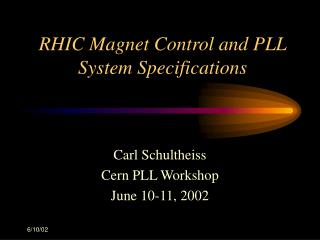DownloadDownload PresentationRHIC Magnet Control and PLL System Specifications

# RHIC Magnet Control and PLL System Specifications

Télécharger la présentation## RHIC Magnet Control and PLL System Specifications

- - - - - - - - - - - - - - - - - - - - - - - - - - - E N D - - - - - - - - - - - - - - - - - - - - - - - - - - -
##### Presentation Transcript

1. RHIC Magnet Control and PLL System Specifications Carl Schultheiss Cern PLL Workshop June 10-11, 2002

2. MAGNET CONTROL SYSTEM Main Quad Bus

3. MAGNET CONTROL SYSTEM Quads at IRs 2,6,8, and 12 o’clock

4. MAGNET CONTROL SYSTEM Waveform Generator Interconnections FEC Waveform Generator Ethernet Serial link to PS RTDL Serial link to PS Event Link

5. MAGNET CONTROL SYSTEM Description of strengths for real-time tune feedback. • Tables of strength versus B for the magnets are calculated by building a ramp using the off-line model. These tables are downloaded to the waveform generators before each ramp.

6. MAGNET CONTROL SYSTEM • The present B is broadcast on the Real Time Data Link (RTDL) to the waveform generators. The waveform generators use the present B to calculate the strength for each magnet.

7. MAGNET CONTROL SYSTEM • Transfer tables of magnet current versus magnet field are also downloaded to the waveform generators. Cubic spline interpolation is used to calculate the required current given the strength for each magnet.

8. MAGNET CONTROL SYSTEM • The quad magnets in the Interaction Regions (IRs) are in the main quad circuit. Their current is individually adjusted by shunting some of the main quad current around them. This is done by nested power supplies.

9. MAGNET CONTROL SYSTEM • The waveform generators subtract the main quad current, and if necessary the trim quad current, from the calculated current. These quad currents are broadcast on the RTDL.

10. MAGNET CONTROL SYSTEM • This current is sent to the power supply interface card. This card has two inputs; the second input is used to send the current of the “previous” waveform generator. This current is subtracted from the total calculated current and the result is sent to the power supply.

11. MAGNET CONTROL SYSTEM • The RTDL also broadcasts delta strengths, in real-time, for the Main Quads and the Sextupoles. This delta strength is added to the strengths from the tables. This adjusts the main quad currents to control the tunes.

12. MAGNET CONTROL SYSTEM • The IR waveform generators subtract the main quad currents from their magnet currents, this compensates the changing main quad currents and the IRs are not disturbed. • The sextupoles can be controlled by a similar process to adjust the chromaticity.

13. PLL SYSTEM SPECIFICATIONS • Specify the damping factor  = 0.7. This is a good compromise in most applications, it provides fast response, small overshoot, and minimum noise bandwidth BL.

14. PLL SYSTEM SPECIFICATIONS • Specify the loop noise bandwidth BL. For stable operation a SNRL should be equal to or greater than 4 (6 dB). Assume a bandpass prefilter with a bandwidth of 500 Hz. and a SNRi of 1 (0 dB) From: the loop noise bandwidth BL is 62.5 Hz.

15. PLL SYSTEM SPECIFICATIONS • The natural frequency of the loop n based on the loop bandwidth and the damping factor is calculated from: and is 897.6 rad/sec. or 142.9 Hz.

16. PLL SYSTEM SPECIFICATIONS • The minimum natural frequency n based on the maximum rate of change of the tune is found from: Assuming a maximum tune rate of change of 0.05 /sec. at a revolution frequency of 77.805 kHz. gives a frequency rate of change of 24443 rad/sec2 The natural frequency must be larger than 221 rad/sec or 35 Hz.

17. PLL SYSTEM SPECIFICATIONS • The two calculations indicate n should be between 35 Hz and 143 Hz. Choose 50 Hz. • In a digital system the sample rate at the compensation filter should be at least 5 times the required bandwidth. This gives a final sample rate of about 250 Hz.

18. PLL SYSTEM SPECIFICATIONS PLL SYSTEM SPECIFICATIONS SUMMARY • The damping factor  = 0.7 • The natural frequency n = 50 Hz • The loop noise bandwidth BL = 26.4 Hz • Compensation Filter Sample Rate = 250Hz • SNRL / SNRi = 18.94 = 25 dB.

19. Qloop Simulation Block Diagram

20. Qloop Simulation

21. Measured Qloop Results

22. Open Loop Qloop Magnitude

23. Closed Loop Qloop Magnitude